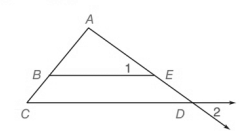Chapter 5.CR, Problem 18CR### Elementary Geometry for College St...

6th Edition
Daniel C. Alexander + 1 other
ISBN: 9781285195698

#### Solutions

Chapter
Section### Elementary Geometry for College St...

6th Edition
Daniel C. Alexander + 1 other
ISBN: 9781285195698
Textbook Problem
1 views

# Given:   ∠ 1 ≅ ∠ 2 .Prove: A B A C = B E C DTo determine

To prove:

ABAC=BECD

Explanation

Given:

12 and

Calculation:

By definition of alternate angles “a pair of angles on the inner side of each of two lines but on opposite sides of the traversal”.

Here, the alternate interior angles are CDE and 2

That is

Then by the theorem,

“If a line is parallel to one side of a triangle and intersects the other two sides, then it divides the sides proportionally”

In the above given figure, ΔACD with BE

### Still sussing out bartleby?

Check out a sample textbook solution.

See a sample solution

#### The Solution to Your Study Problems

Bartleby provides explanations to thousands of textbook problems written by our experts, many with advanced degrees!

Get Started

#### Find more solutions based on key concepts